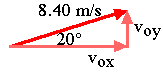#### Projectile Motion Example

Let's say you're on top of a cliff, which drops vertically 150 m to the ocean below. You throw a ball with an initial speed of 8.40 m/s at an angle of 20 degrees above the horizontal.

(a) How long does it take before it hits the water?

(b) How far is it from the base of the cliff to the point of impact?

As usual, be as systematic as possible. Draw a diagram, choose a coordinate system, and organize your data.X Info. Y Info.
Initial positionxo = 0 yo = +150 m
Final position x = ? y = 0
Initial velocity vox= +vo cos(q)
= +7.893 m/s
voy= +vo sin(q)
= +2.873 m/s
Acceleration ax = 0 ay = -9.8 m/s2

#### Part (a)

(a) How long does it take before it hits the water?

Use the y-information to find the time of flight. One method is to do it in two steps, first calculating the final y-velocity using the equation:

vy 2 = voy2 + 2 ay (y - yo)

This gives vy 2 = 2.8732 + 2 (-9.8) (-150) = 2948.3 m2 / s2 .
Taking the square root gives: vy = +/- 54.30 m/s.

Remember that the square root can be positive or negative. In this case it's negative, because the y-component of the velocity will be directed down when the ball hits the ground.

Now find the time using:

vy = voy + ay t

So, -54.30 = 2.873 - 9.8 t, which gives t = 5.834 seconds.
Rounding off, the ball was in the air for 5.83 s.

#### Part (b)

(b) How far is it from the base of the cliff to the point of impact?

Plug the time we just calculated into the equation:

x - xo = vox t + ˝ ax t2

x = (7.893) (5.834) = 46.0 m.